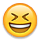## Forum Stats

• 3,757,576 Users
• 2,251,248 Discussions

Discussions

# query

HI all,
I have atable with 25 columns.
a b -------------y
no yes no
no yes yes
yes no no

I have to calculate how many yes and no are there in each column.The output should be
status a b----------------upto y
NO 2 1 2
YES 1 2 1

How can iachieve this in single query?

THanks

• Just the examples for two-columns case:
```SQL> with t as (
2   select 'y' a, 'n' b from dual
3   union all
4   select 'n' a, 'y' b from dual
5   union all
6   select 'n' a, 'y' b from dual
7   union all
8   select 'n' a, 'y' b from dual
9   union all
10   select 'y' a, 'y' b from dual
11   union all
12   select 'n' a, 'n' b from dual
13   union all
14   select 'y' a, 'y' b from dual
15   union all
16   select 'y' a, 'n' b from dual
17  )
18  select 'YES' status,sum(decode(a,'y',1)) a, sum(decode(b,'y',1)) b
19  from t
20  union all
21  select 'NO',sum(decode(a,'n',1)), sum(decode(b,'n',1))
22  from t
23  /
STA          A          B
--- ---------- ----------
YES          4          5
NO           4          3
SQL> with t as (
2   select 'y' a, 'n' b from dual
3   union all
4   select 'n' a, 'y' b from dual
5   union all
6   select 'n' a, 'y' b from dual
7   union all
8   select 'n' a, 'y' b from dual
9   union all
10   select 'y' a, 'y' b from dual
11   union all
12   select 'n' a, 'n' b from dual
13   union all
14   select 'y' a, 'y' b from dual
15   union all
16   select 'y' a, 'n' b from dual
17  )
18  select decode(rn,1,'YES','NO') status,
19  decode(rn,1,a_y,a_n) "A",
20  decode(rn,1,b_y,b_n) "B"
21  from
22  (
23  select sum(decode(a,'y',1)) a_y,
24  sum(decode(a,'n',1)) a_n,
25  sum(decode(b,'y',1)) b_y,
26  sum(decode(b,'n',1)) b_n
27  from t
28  ) arrg,
29  (select rownum rn from dual connect by level <=2) pivot
30  /
STA          A          B
--- ---------- ----------
YES          4          5
NO           4          3```
Of course, for 25 columns the extentions of examples will be very complicated.

Rgds.
• I recommecd using "model clause"```with t as(
select 'y' a, 'n' b from dual union all
select 'n' a, 'y' b from dual union all
select 'n' a, 'y' b from dual union all
select 'n' a, 'y' b from dual union all
select 'y' a, 'y' b from dual union all
select 'n' a, 'n' b from dual union all
select 'y' a, 'y' b from dual union all
select 'y' a, 'n' b from dual)
select soeji,cntA,cntB
from t
model return updated rows
dimension by(cast(RowNum as varchar2(3)) as soeji)
measures(a,b,0 as cntA,0 as cntB)
rules(
cntA['yes'] = count(decode(a,'y',1))[any],
cntB['yes'] = count(decode(b,'y',1))[any],
cntA['no']  = count(decode(a,'n',1))[any],
cntB['no']  = count(decode(b,'n',1))[any]);

SOE  CNTA  CNTB
---  ----  ----
yes     4     5
no      4     3```
This discussion has been closed.